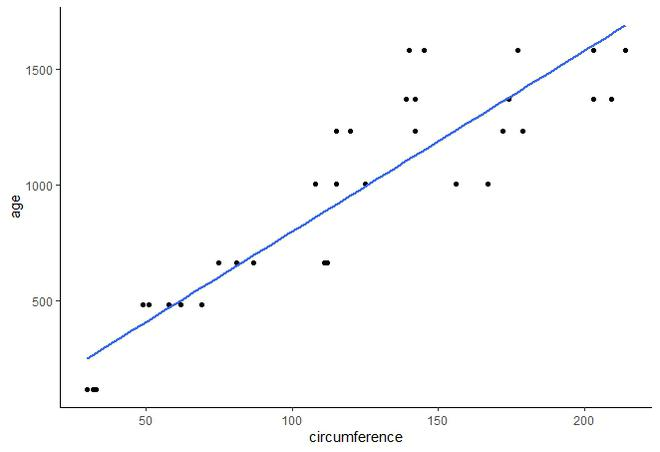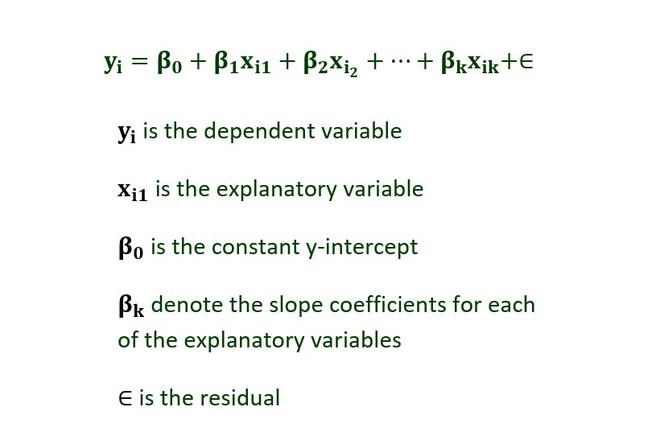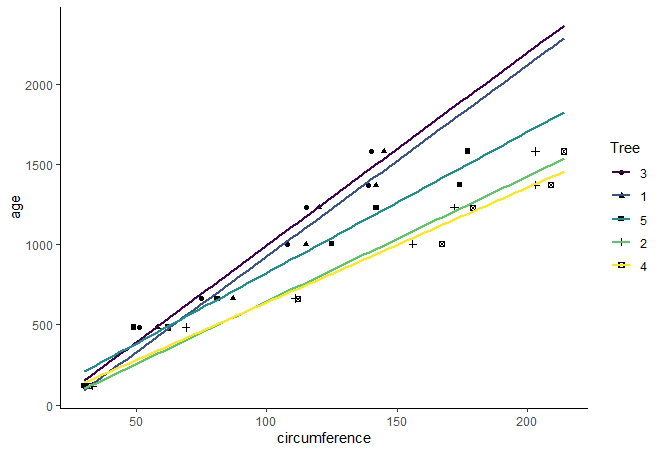Multiple linear regression using ggplot2 in R

• Last Updated : 24 Jun, 2021

A regression line is basically used in statistical models which help to estimate the relationship between a dependent variable and at least one independent variable. There are two types of regression lines :

• Single Regression Line.
• Multiple Regression Lines.

In this article, we are going to discuss how to plot multiple regression lines in R programming language using ggplot2 scatter plot.

Dataset Used: Here we are using a built-in data frame “Orange” which consists of details about the growth of five different types of orange trees. The data frame has 35 rows and 3 columns. The columns in this data frame are :

• Tree: The ordering of trees on which experiment is made on the basis of increasing diameter values of the orange.
• Age: The age of the trees since when they were planted.
• Circumference: The circumference of the orange.

We first create a scatter plot. We will use the function geom_point( ) to plot the scatter plot which comes under the ggplot2 library.

Syntax:

geom_point( mapping=NULL, data=NULL, stat=identity, position=”identity”)

Basically, we are doing a comparative analysis of the circumference vs age of the oranges. The function used is geom_smooth( ) to plot a smooth line or regression line.

Syntax: geom_smooth(method=”auto”,se=FALSE,fullrange=TRUE,level=0.95)

Parameter :

• method : The smoothing method is assigned using the keyword loess, lm, glm etc
• lm : linear model, loess : default for smooth lines during small data set observations.
• formula : You can also use formulas for smooth lines. For example : y~poly(x,4) which will plot a smooth line of degree 4. Higher the degree more bends the smooth line will have.
• se : It takes logical values either “TRUE” or “FALSE”.
• fullrange : It takes logical value either “TRUE” or “FALSE”.
• level : By default level is 0.95 for the confidence interval.

Let us first draw a simple single-line regression and then increase the complexity to multiple lines.

Example:

R

 # Scatter Plotlibrary(ggplot2)  ggplt <- ggplot(Orange,aes(x=circumference,y=age))+         geom_point()+         theme_classic()  ggplt  # Plotting a single Regression Lineggplt+geom_smooth(method=lm,se=FALSE,fullrange=TRUE)

Output:This is a single smooth line or popularly known as a regression line. Here, the points are combined and are not segregated on the basis of any groups.

Multiple linear regression will deal with the same parameter, but each line will represent a different group. So, if we want to plot the points on the basis of the group they belong to, we need multiple regression lines. Each regression line will be associated with a group.

Basic Formula for Multiple Regression Lines :The syntax in R to calculate the coefficients and other parameters related to multiple regression lines is :

var <- lm(formula, data = data_set_name)

summary(var)

lm : linear model

var : variable name

To compute multiple regression lines on the same graph set the attribute on basis of which groups should be formed to shape parameter.

Syntax:

shape = attribute

A single regression line is associated with a single group which can be seen in the legends of the plot. Now, to assign different colors to every regression lines write the command :

color = attribute

Example:

R

 library(ggplot2)  ggplt <- ggplot(Orange,aes(x=circumference,y=age,shape=Tree))+         geom_point()+         theme_classic()  ggplt  # Plotting multiple Regression Linesggplt+geom_smooth(method=lm,se=FALSE,fullrange=TRUE,                  aes(color=Tree))

Output:My Personal Notes arrow_drop_up